Starts With A Bang

# This Is Why The Speed Of Gravity Must Equal The Speed Of Light

#### It’s been spectacularly confirmed by observation, but theoretically, it couldn’t have been any other way.

If the Sun were to spontaneously cease emitting light, we wouldn’t know about it for about 8 minutes and 20 seconds. The light that’s arriving here on Earth, right at this very moment, was emitted from the Sun’s photosphere a finite amount of time in the past, and is only being seen now after a journey across the 150 million km (93 million miles) separating the Sun from the Earth. If the Sun were to go dark right now, we wouldn’t find out until the light stopped arriving.

But what about gravitationally? If the Sun were spontaneously (somehow) removed from existence, how long would Earth remain in its elliptical orbit before flying off in a straight line? Believe it or not, the answer to this must be exactly the same amount of time as it was for light: 8 minutes and 20 seconds. The speed of gravity not only equals the speed of light to an incredibly precise degree observationally, but these two constants must be exactly equal theoretically, or General Relativity would fall apart. Here’s the science behind why.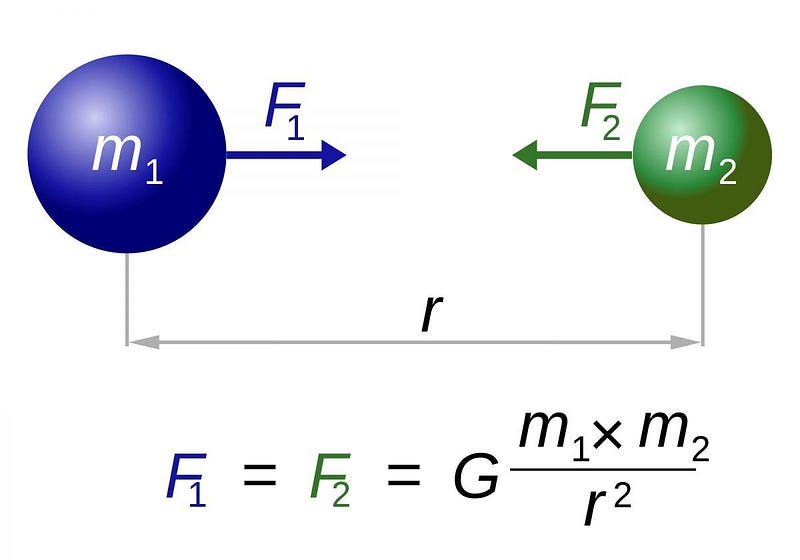Newton’s law of Universal Gravitation has been superseded by Einstein’s General Relativity, but relied on the concept of an instantaneous action (force) at a distance, and is incredibly straightforward. The gravitational constant in this equation, G, along with the values of the two masses and the distance between them, are the only factors in determining a gravitational force. G also appears in Einstein’s theory. (WIKIMEDIA COMMONS USER DENNIS NILSSON)

Before General Relativity came along, our most successful theory of gravity was Newton’s universal law of gravitation. According to Newton, the gravitational force between any two objects in space defined by just four parameters:

1. The gravitational constant of the Universe, G, which is the same for everyone.
2. The mass of the first object, m, which experiences the gravitational force. (By Einstein’s equivalence principle, this is the same m that goes into the laws of motion, like F = ma.)
3. The mass of the second object, M, which attracts the first object.
4. The distance between them, r, which extends from the center-of-mass of the first object to the center-of-mass of the second.

Keep in mind that these are the only four parameters that are allowable in Newtonian gravitation. You can perform all sorts of calculations from this force law to derive, for example, elliptical planetary orbits around the Sun. But the equations only work if the gravitational force is instantaneous.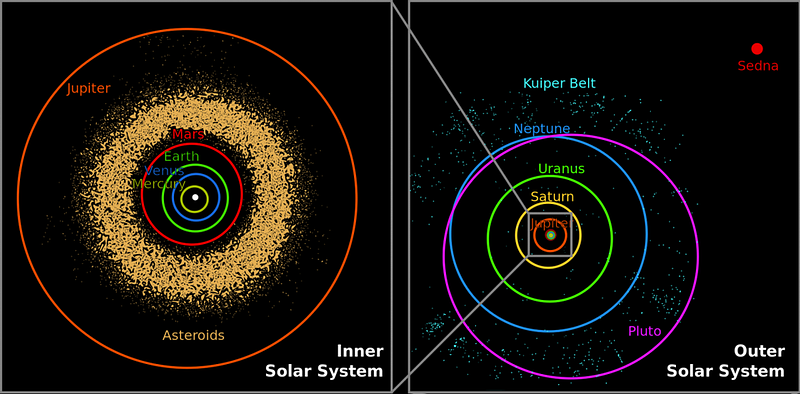The orbits of the eight major planets vary in eccentricity and the difference between perihelion (closest approach) and aphelion (farthest distance) with respect to the Sun. There is no fundamental reason why some planets are more or less eccentric than one another; it’s simply a result of the initial conditions from which the Solar System formed. However, if you were to somehow turn ‘off’ the gravitational effects of the Sun, the planets wouldn’t fly off instantaneously, but rather the inner ones would fly off first, followed by the outer ones, as the gravitational signals from the Sun only propagate outwards at the speed of gravity, which ought to equal the speed of light. (NASA / JPL-CALTECH / R. HURT)

This might puzzle you a little bit. After all, if the speed of gravity is only equal to the speed of light, rather than an infinitely fast force, then the Earth should be attracted to where the Sun was 8 minutes and 20 seconds ago, not where the Sun is right now, at this particular instant in time. But if you do that calculation instead, and allow Earth to be attracted to the Sun’s past position rather than its current position, you get a prediction for its orbit that is so thoroughly wrong that Newton himself, with quality observations going back less than 100 years (to the time of Tycho Brahe), could have ruled it out.

In fact, if you used Newton’s laws to calculate the orbits of the planets and demanded that they match modern observations, not only would the speed of gravity have to be faster than the speed of light, it would have to be a minimum of 20 billion times faster: indistinguishable from an infinite speed.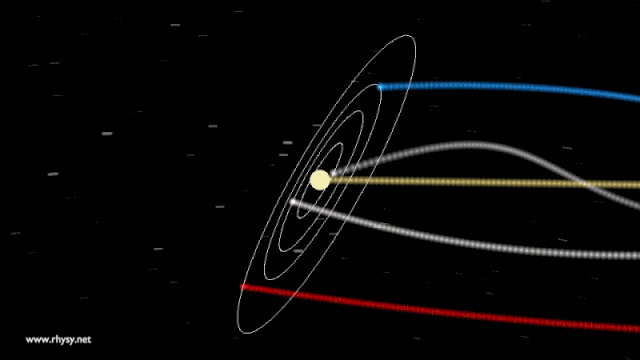An accurate model of how the planets orbit the Sun, which then moves through the galaxy in a different direction-of-motion. If the Sun were to simply wink out of existence, Newton’s theory predicts that they would all instantaneously fly off in straight lines, while Einstein’s predicts that the inner planets would continue orbiting for shorter periods of time than the outer planets. (RHYS TAYLOR)

The problem is this: if you have a central force, where a bound particle like (for example) the Earth is attracted to the Sun but moves around the Sun (orbiting, or propagating) at a finite speed, you will only get a purely elliptical orbit if that force’s propagation speed is infinite. If it’s finite, then you don’t just get a radial acceleration (towards the other mass), but you also get a component that accelerates your particle tangentially.

This would make orbits not only elliptical, but unstable. On the scale of a mere century, orbits would shift substantially. By 1805, Laplace had used observations of the Moon to demonstrate that the speed of Newtonian gravity must be 7 million times greater than the speed of light. Modern constraints are now 20 billion times the speed of light, which is great for Newton. But all of this placed a great burden on Einstein.One revolutionary aspect of relativistic motion, put forth by Einstein but previously built up by Lorentz, Fitzgerald, and others, that rapidly moving objects appeared to contract in space and dilate in time. The faster you move relative to someone at rest, the greater your lengths appear to be contracted, while the more time appears to dilate for the outside world. This picture, of relativistic mechanics, replaced the old Newtonian view of classical mechanics, but also carries tremendous implications for theories that aren’t relativistically invariant, like Newtonian gravity. (CURT RENSHAW)

According to Einstein, there’s a big problem, conceptually, with Newton’s gravitational force law: the distance between any two objects is not an absolute quantity, but rather is dependent on the motion of the observer. If you’re moving towards or away from any imaginary line you draw, distances in that direction will contract, depending on your relative velocities. For the gravitational force to be a calculable quantity, all observers would have to derive consistent results, something that you cannot get by combining relativity with Newton’s gravitational force law.

Therefore, according to Einstein, you’d have to develop a theory that brought gravitation and relativistic motions together, and that meant developing General Relativity: a relativistic theory of motion that incorporated gravity into it. Once completed, General Relativity told a dramatically different story.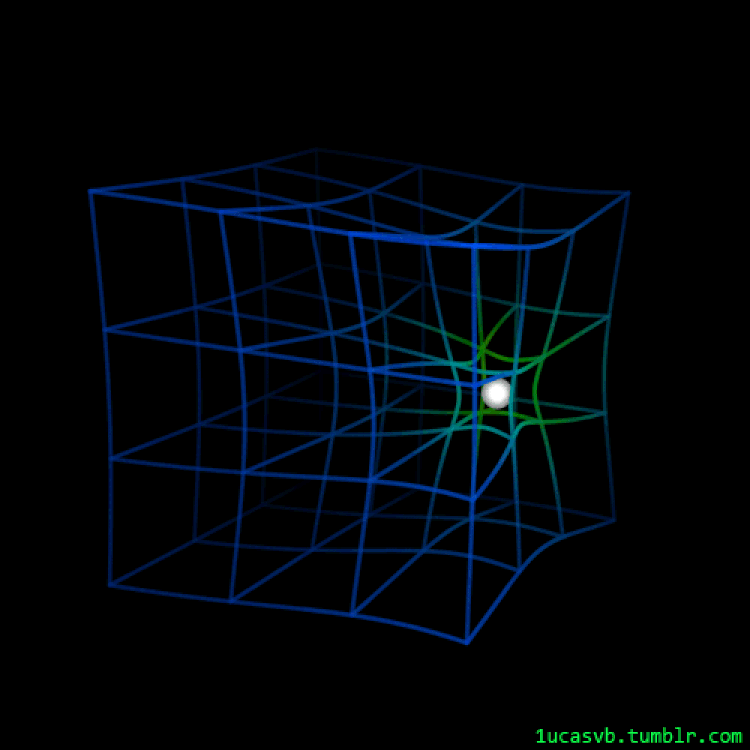An animated look at how spacetime responds as a mass moves through it helps showcase exactly how, qualitatively, it isn’t merely a sheet of fabric but all of space itself gets curved by the presence and properties of the matter and energy within the Universe. Note that spacetime can only be described if we include not only the position of the massive object, but where that mass is located throughout time. Both instantaneous location and the past history of where that object was located determine the forces experienced by objects moving through the Universe. (LUCASVB)

In order to get different observers to agree on how gravitation works, there can be no such thing as absolute space, absolute time, or a signal that propagates at infinite speed. Instead, space and time must both be relative for different observers, and signals can only propagate at speeds that exactly equal the speed of light (if the propagating particle is massless) or at speeds that are below the speed of light (if the particle has mass).

In order for this to work out, though, there has to be an additional effect to cancel out the problem of a non-zero tangential acceleration, which is induced by a finite speed of gravity. This phenomenon, known as gravitational aberration, is almost exactly cancelled by the fact that General Relativity also has velocity-dependent interactions. As the Earth moves through space, for example, it feels the force from the Sun change as it changes its position, the same way a boat traveling through the ocean will come down in a different position as it gets lifted up and lowered again by a passing wave.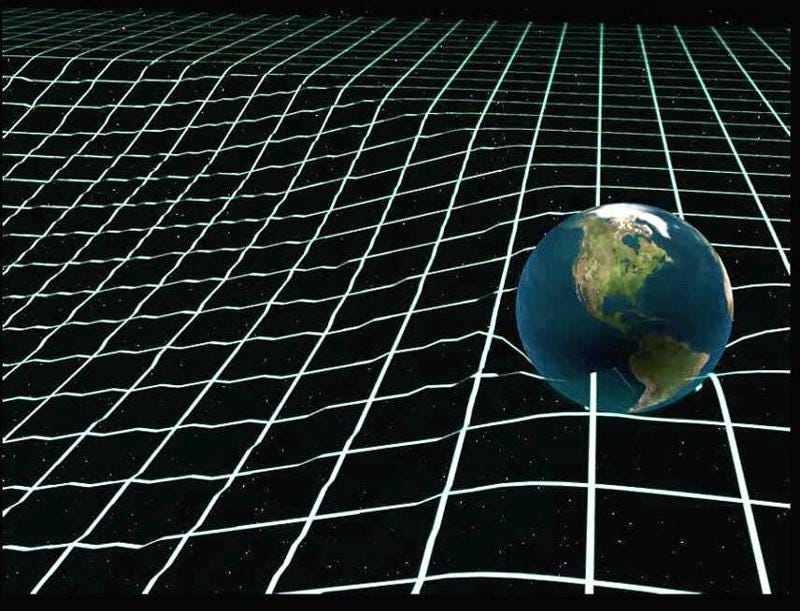Gravitational radiation gets emitted whenever a mass orbits another one, which means that over long enough timescales, orbits will decay. Before the first black hole ever evaporates, the Earth will spiral into whatever’s left of the Sun, assuming nothing else has ejected it previously. Earth is attracted to where the Sun was approximately 8 minutes ago, not to where it is today. (AMERICAN PHYSICAL SOCIETY)

What’s remarkable, and by no means obvious, is that these two effects cancel almost exactly. The fact that the speed of gravity is finite is what induces this gravitational aberration, but the fact that General Relativity (unlike Newtonian gravity) has velocity-dependent interactions is what allowed Newtonian gravity to be such a good approximation. There’s only one speed that works to make this cancellation a good one: if the speed of gravity equals the speed of light.

So that’s the theoretical motivation for why the speed of gravity should equal the speed of light. If you want planetary orbits to be consistent with what we’ve seen, and to be consistent for all observers, you need a speed of gravity that equals c, and to have your theory be relativistically invariant. There’s another caveat, however. In General Relativity, the cancellation between the gravitational aberration and the velocity-dependent term is almost exact, but not quite. Only the right system can reveal the difference between Einstein’s and Newton’s predictions.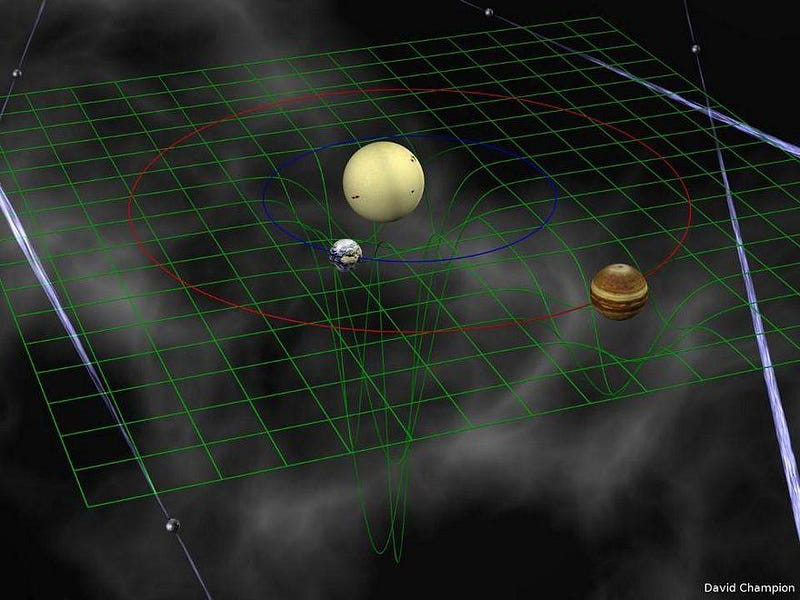When a mass moves through a region of curved space, it will experience an acceleration owing to the curved space it inhabits. It also experiences an additional effect due to its velocity as it moves through a region where the spatial curvature is constantly changing. These two effects, when combined, result in a slight, tiny difference from the predictions of Newton’s gravity. (DAVID CHAMPION, MAX PLANCK INSTITUTE FOR RADIO ASTRONOMY)

In our own neighborhood, the force of the Sun’s gravity is far too weak to produce a measurable effect. What you’d want is a system that had large gravitational fields at small distances from a massive source, where the velocity of the moving object is both fast and changing (accelerating) rapidly, in a gravitational field with a large gradient.Travel the Universe with astrophysicist Ethan Siegel. Subscribers will get the newsletter every Saturday. All aboard!

Our Sun doesn’t give us that, but the environment around either a binary black hole or a binary neutron star does! Ideally, a system with a massive object moving with a changing velocity through a changing gravitational field will showcase this effect. And a binary neutron star system, where one of the neutron stars is a very precise pulsar, fits the bill exactly.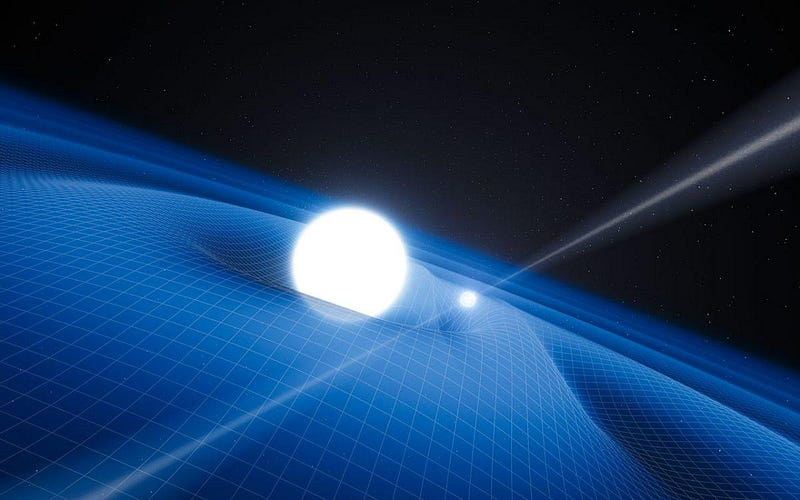When you have a single object, like a pulsar, orbiting in space, it will pulse every time it completes a 360 degree rotation to a fortuitously aligned observer. If you place that pulsar in a binary system with another dense, massive object, it will move quickly through that space, exhibiting both the effects of gravitational aberration and velocity-dependent interactions, and their inexact cancellation allows scientists to discern the relativistic predictions for this system from the Newtonian ones. (ESO/L. CALÇADA)

A pulsar, and in particular, a millisecond pulsar, is the best natural clock in the Universe. As the neutron star spins, it emits a jet of electromagnetic radiation that has a chance of being aligned with Earth’s perspective once every 360 degree rotation. If the alignment is right, we’ll observe these pulses arriving with extraordinarily predictable accuracy and precision.

If the pulsar is in a binary system, however, then moving through that changing gravitational field will cause the emission of gravitational waves, which carry energy away from the gravitating system. The loss of that energy has to come from somewhere, and is compensated by the decay of the pulsar’s orbits. The predictions of pulsar decay is highly sensitive to the speed of gravity; using even the very first binary pulsar system ever discovered by itself, PSR 1913+16 (or the Hulse-Taylor binary), allowed us to constrain the speed of gravity to be equal to the speed of light to within only 0.2%!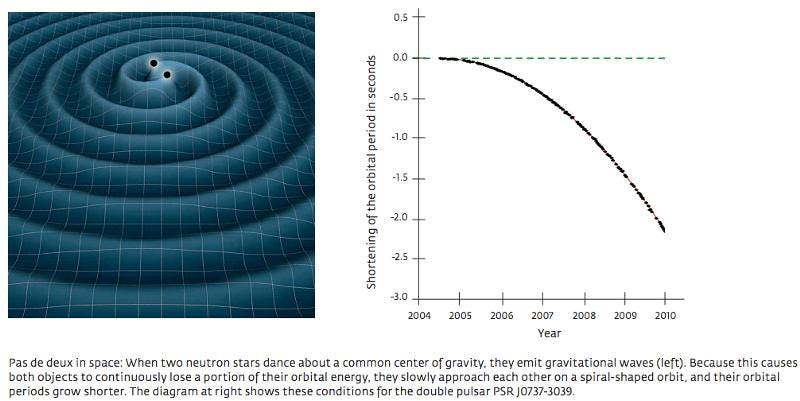The rate of orbital decay of a binary pulsar is highly dependent on the speed of gravity and the orbital parameters of the binary system. We have used binary pulsar data to constrain the speed of gravity to be equal to the speed of light to a precision of 99.8%, and to infer the existence of gravitational waves decades before LIGO and Virgo detected them. However, the direct detection of gravitational waves was a vital part of the scientific process, and the existence of gravitational waves would still be in doubt without it. (NASA (L), MAX PLANCK INSTITUTE FOR RADIO ASTRONOMY / MICHAEL KRAMER (R))

Since that time, other measurements have also demonstrated the equivalence between the speed of light and the speed of gravity. In 2002, chance coincidence caused the Earth, Jupiter, and a very strong radio quasar (known as QSO J0842+1835) to all align. As Jupiter passed between the Earth and the quasar, its gravitational effects caused the starlight to bend in a fashion that was speed-of-gravity dependent.

Jupiter did, in fact, bend the light from the quasar, enabling us to rule out an infinite speed for the speed of gravity and determine that it was actually between 255 million and 381 million meters-per-second, consistent with the exact value for the speed of light (299,792,458 m/s) and also with Einstein’s predictions. Even more recently, the first observations of gravitational waves brought us even tighter constraints.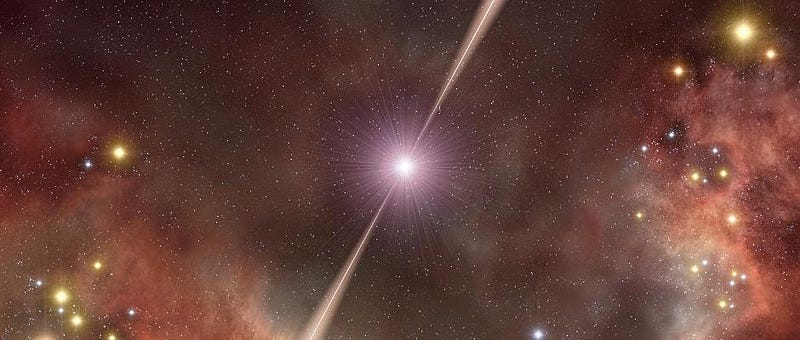Illustration of a fast gamma-ray burst, long thought to occur from the merger of neutron stars. The gas-rich environment surrounding them could delay the arrival of the signal, explaining the observed 1.7 second difference between the arrivals of the gravitational and electromagnetic signatures. This is the best evidence we have, observationally, that the speed of gravity must equal the speed of light. (ESO)

From the very first gravitational wave detected and the difference in their arrival times at Hanford, WA and Livingston, LA, we directly learned that the speed of gravity equaled the speed of light to within about 70%, which isn’t an improvement over the pulsar timing constraints. But when 2017 saw the arrival of both gravitational waves and light from a neutron star-neutron star merger, the fact that gamma-ray signals came just 1.7 seconds after the gravitational wave signal, across a journey of over 100 million light years, taught us that the speed of light and the speed of gravity differ by no more than 1 part in a quadrillion: 10¹⁵.

As long as gravitational waves and photons have no rest mass, the laws of physics dictate that they must move at exactly the same speed: the speed of light, which must equal the speed of gravity. Even before the constraints got this spectacular, requiring that a gravitational theory reproduce Newtonian orbits while simultaneously being relativistically invariant leads to this inevitable conclusion. The speed of gravity is exactly the speed of light, and physics wouldn’t have allowed it to be any other way.

Ethan Siegel is the author of Beyond the Galaxy and Treknology. You can pre-order his third book, currently in development: the Encyclopaedia Cosmologica.

Related
More work is needed before declaring the technique a fountain of youth.
Hinduism emphasizes the journey, whichever path that takes. And it holds us responsible for our own self-improvement.
An unexpected ancient manufacturing strategy may hold the key to designing concrete that lasts for millennia.
Quantum superposition challenges our notions of what is real.
The benefits of employee training are felt far and wide, from improvements in workers’ wellbeing to better customer interactions.

Up Next
Unnatural Selection and the thrilling, terrifying possibilities of genetic editing.